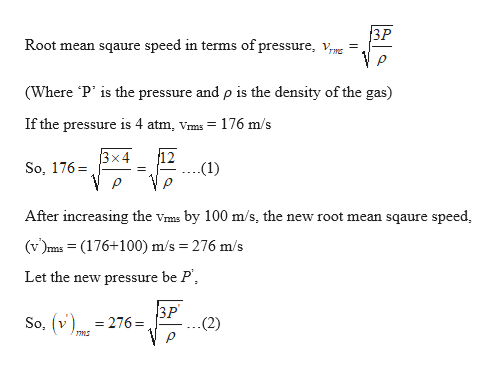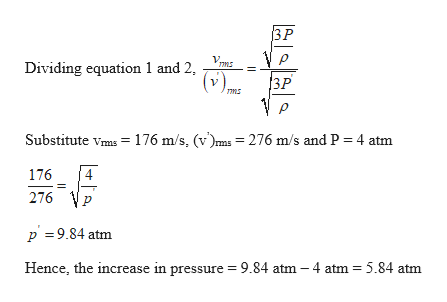Helium gas is in a cylinder that has rigid walls. If the pressure of the gas is 4.00 atm, then the root-mean-square speed of the helium atoms is Vrms = 176 m/s.By how much (in atmospheres) must the pressure be increased to increase the Vrms of the He atoms by 100 m/s? Ignore any change in the volume of the cylinder. Δp = __________ atm

Question

Helium gas is in a cylinder that has rigid walls. If the pressure of the gas is 4.00 atm, then the root-mean-square speed of the helium atoms is Vrms = 176 m/s.

By how much (in atmospheres) must the pressure be increased to increase the Vrms of the He atoms by 100 m/s? Ignore any change in the volume of the cylinder.

Δp = __________ atm

Step 1

Given:

RMS speed of the helium gas at 4 atm pressure = 176 m/s

Step 2

Calculating the new root mean square speed:help_outlineImage Transcriptionclose3P Root mean sqaure speed in terms of pressure, vmg = (Where P' is the pressure and p is the density of the gas) If the pressure is 4 atm, Vms 176 m/s 12 (1) x4 So, 176 After increasing the vms by 100 m/s, the new root mean sqaure speed (v)s = (176+100) m/s 276 m/s Let the new pressure be P 3P .(2) So, v 276 ms fullscreen
Step 3

Calculating the change in pressure to in...help_outlineImage Transcriptionclose3P VP 3P ms Dividing equation 1 and 2, (v) Substitute vmas 176 m/s, (v')ms = 276 m/s and P 4 atm 4 176 276 p 9.84 atm Hence, the increase in pressure 9.84 atm - 4 atm 5.84 atm fullscreen

Want to see the full answer?

See Solution

Want to see this answer and more?

Our solutions are written by experts, many with advanced degrees, and available 24/7

See Solution
Tagged in

Kinetic Theory of Gases